Markets
Futures
Earn
More
Cancel
FAQAccount FunctionsTutorialBinance Fan TokenBinance EarnCrypto Deposit/Withdrawal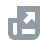Crypto Derivatives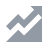FinanceAPISecurityOther Topics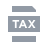Tax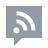Feed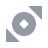Binance Convert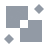NFTVIP
Home
Support Center
FAQ
Crypto Derivatives
Futures Contracts
Multi-Assets Mode
How to Calculate Margin in Multi-Assets Mode

# How to Calculate Margin in Multi-Assets Mode

2022-07-06 07:02

## How to check your account margin ratio under the Multi-Assets Mode?

If you select the [Multi-Assets Mode], you will see a [Multi-Assets] tag on top of the Margin Ratio widget.
Since your margin balance is shared across multiple positions in the Multi-Assets Mode, please monitor your margin ratio closely. Once the margin ratio reaches 100%, all positions will be liquidated.

## How to calculate the margin ratio in the Multi-Assets Mode?

You can use the following equation to calculate your margin ratio in the Multi-Assets Mode:
Account Margin Ratio = Account Maintenance Margin / Account Equity
• Account Maintenance Margin: Sum of the Maintenance Margin of all cross positions (in USD).
• Account Equity: Sum of margin balance of all cross positions (in USD).
Please note that the margin ratio is based on the prevailing market rate for USDTUSD and BUSDUSD pairs.
Example:
Suppose the asset pairs and their respective floating conversion rates are as follows:
You want to trade two contracts, BTCUSDT perpetual and ETHBUSD_210326 with leverage of 100x and 50x respectively. In this scenario, the Maintenance Margin Ratio will be 0.8% and 1% respectively. Whether you are using the Isolated or the Cross Margin Mode, the account status and calculation changes of various parameters are as follows:

### 1. Initial state - No open positions

Under the Single-Asset Mode:
USDT available for order = 200 BUSD available for order = 220
Under the Multi-Assets Mode:
Account Equity = ∑min(assetEquity*bidRate, assetEquity*askRate) = 200 * 0.9801 + 220 * 1 = 416.02
As there are no open positions, assetCrossInitialMargin = 0
uniAvailableForOrder = accountEquity-∑(assetCrossInitialMargin*askRate) = 416.02 - 0 = 416.02
USDT Available for Order = 416.02 / 0.99495 = 418.13
BUSD Available for Order = 416.02 / 1 = 416.02
Account Maintenance Margin Ratio = 0
Margin Ratio = Account Maintenance Margin / Account Equity = 0

### 2. With open positions

Account Maintenance Margin = ∑assetMM*askRate = 0.5 * 20,000 * 0.008 * 0.99495 + 20 * 600 * 0.01 * 1 = 199.596
As there is no unrealized PnL, Account Equity = accountWalletBalance = 416.02
uniAvailableForOrder = Account Equity - ∑(assetCrossInitialMargin * askRate) = 416.02 - (0.5 * 20,000 * 0.01 * 0.99495 + 20 * 600 * 0.02 * 1)= 76.525
USDT Available for Order = 76.525 / 0.99495 = 76.91
BUSD Available for Order = 76.525 / 1 = 76.525
Margin Ratio = Account Maintenance Margin / Account Equity = 199.596 / 416.02 = 0.47977 = 47.98%

### 3. With open positions and unrealized PnL

Account Maintenance Margin = ∑assetMM*askRate = 0.5 * 19,000 * 0.008 * 0.99495 + 20 * 620 * 0.01 * 1 = 199.61
With unrealized PnL, Account Equity = ∑min(assetEquity*bidRate, assetEquity*askRate) = -300 * 0.99495 + 620 * 1 = 321.515
uniAvailableForOrder = Account Equity - ∑(assetCrossInitialMargin * askRate) = 321.515 - (0.5 * 19,000 * 0.01 * 0.99495 + 20 * 620 * 0.02 * 1) = -21
USDT Available for Order and BUSD Available for Order are both 0
Margin Ratio = Account Maintenance Margin / Account Equity = 199.61 / 321.515 = 0.62084 = 62.08%